Normal view

# Scattering Theory (Methods of Modern Mathematical Physics, Vol. 3).

Publisher: 1979ISBN: 0125850034; 9780125850032DDC classification: 530.15
Tags from this library: No tags from this library for this title.Average rating: 0.0 (0 votes)
Item type Current location Collection Call number Status Date due Barcode Item holdsBook Books at IST Austria
Erdős Group 530.15 (Browse shelf) Not for loanBook Library
53x (Browse shelf) Available AT-ISTA#000459
Total holds: 0
##### Close shelf browser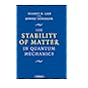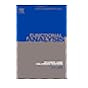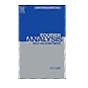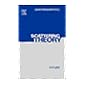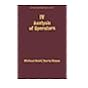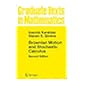53x The Stability of Matter in Quantum Mechanics. 53x I: Functional Analysis, Volume 1 (Methods of Modern Mathematical Physics) (vol 1). 53x Fourier Analysis, Self-Adjointness (Methods of Modern Mathematical Physics, Vol. 2). 53x Scattering Theory (Methods of Modern Mathematical Physics, Vol. 3). 53x IV: Analysis of Operators, Volume 4 (Methods of Modern Mathematical Physics). 53x Turbulent Flows. 53x Brownian Motion and Stochastic Calculus (Graduate Texts in Mathematics).

There are no comments for this item.